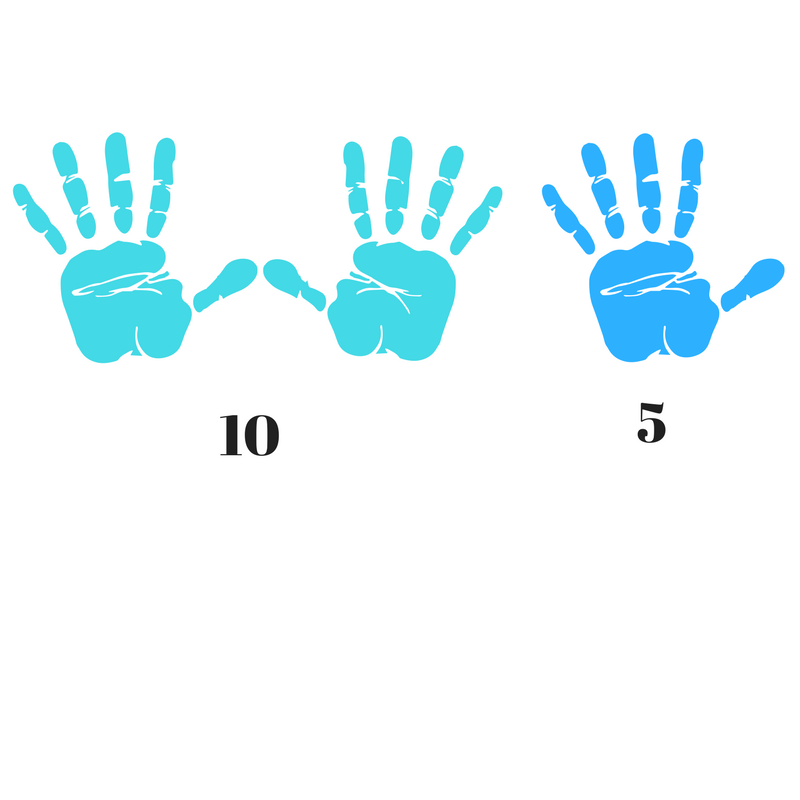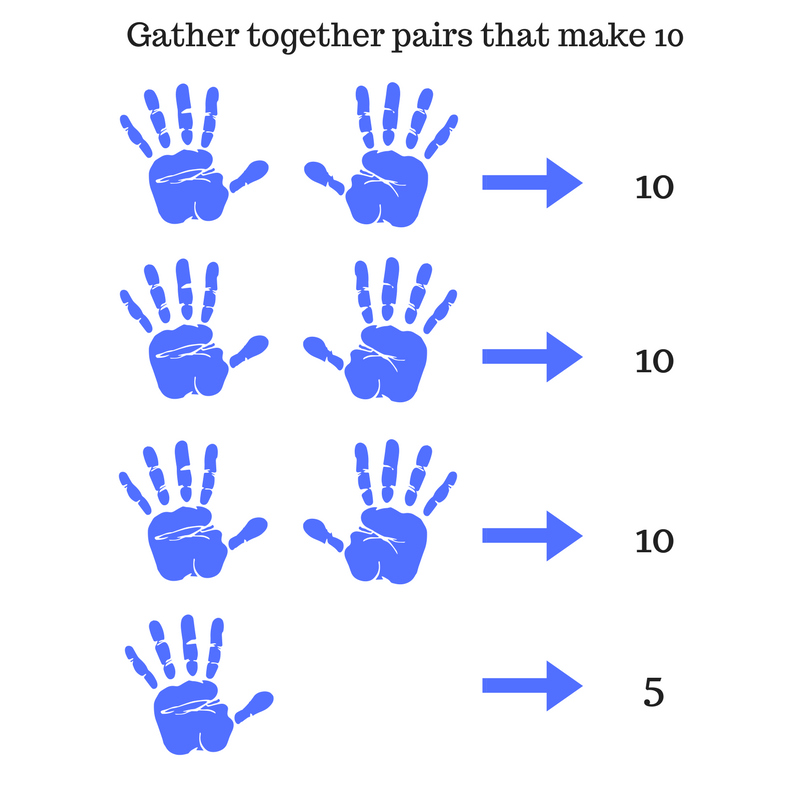Search

# 5x table memory ideas

Stuck for how to remember the 5x table?

It is a great times table for younger learners as (a) we have five fingers on each hand, which really helps with visualising what is going on and (b) 5 is half of ten. So if you multiply a number by 10, you can halve your answer to find that number multiplied by 5.

1 x 5 = 5

Speak this to yourself and read the "x" sign as " lot of".

One lot of 5 is 5.A most useful feature of the 5x table is that multiples of 5 can easily be dealt with by being grouped in pairs to make groups of 10

2x5 = 10

Two lots of five3x5 = 15

Three lots of five4x5 = 20

Think fingers and toes to make up your four lots of 5.

Group 5 and 5 to make 10

Two lots of 10 make 205x5 = 25

Try a rhyme using this image from Kippson.

The shape of the number 5 looks like the letter "S" so instead of 5 x 5 we get SS (Sally Skydiver).

"Sally Skydiver's 25. She is glad to be alive"6x5 = 30

There are five minutes between each of the numbers marked on a clock face.

6 lots of 5 occurs at the bottom of the clock face when it is 30 minutes past the hour7x5 = 35

7 lots of 5 can sound daunting

Try gathering the groups of 5 together to make groups of 10.

8x5 = 40

Gather pairs, as above (you will get 4 groups of 10)

Alternatively, as with any even number group of 5, firstly multiply by 10, then divide your answer in half

8 x 10 = 80

so 8 x 5 is half that number (80 divided by 2 is 40)9x5 = 45

You may be more familiar with your 10x tables?

If so,

10 lots of 5 is 50

You can count backwards from here.

9 lots of five must be 5 less than 50

ie 50 - 5 = 45If you are using Kippson's flash cards or stickers, a little rhyme can help

"9 x 5 is eagle-eyed"

To add to the imagery, the "4" is like the sharp beak of the bird, and the 5 could be a wiggly worm

10x5 = 50

The digits of any number multiplied by 10 will all move one column to the left

5 becomes 5011x5 = 55

Think of 11 lots of 5 as being 10 lots of 5 plus another 5

10 x 5 = 50, so 11 lots of 5 is 50 +512x5 = 60

There are five minutes between each of the numbers marked on a clock face.

12 lots of 5 occurs at the top of the clock face when it is 60 minutes past the hour

For more multiplication learning ideas, worksheets and reference sheets see Kippson's free resources page

See All